Anzeige

# Chapter two

21. Jul 2021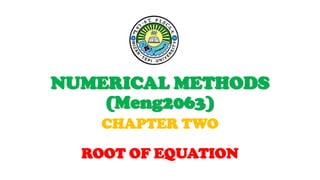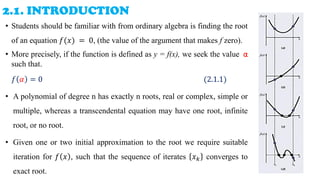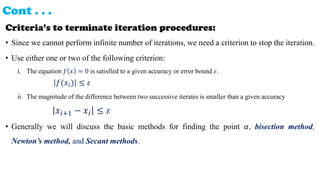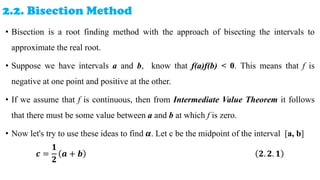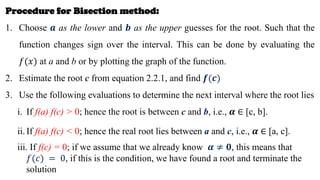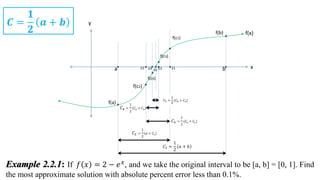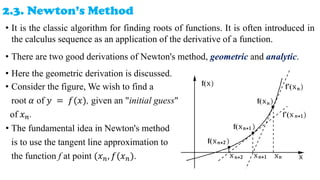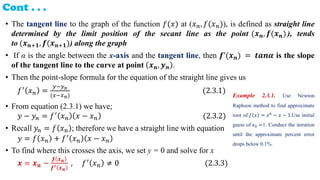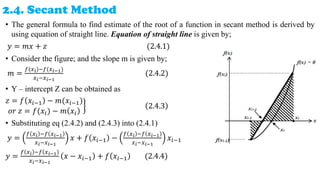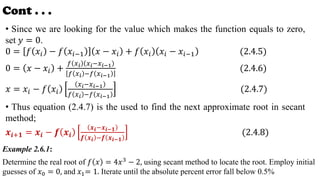1 von 11
Anzeige

### Chapter two

1. NUMERICAL METHODS (Meng2063) CHAPTER TWO ROOT OF EQUATION
2. 2.1. INTRODUCTION • Students should be familiar with from ordinary algebra is finding the root of an equation 𝑓(𝑥) = 0, (the value of the argument that makes f zero). • More precisely, if the function is defined as y = f(x), we seek the value α such that. 𝑓 𝛼 = 0 (2.1.1) • A polynomial of degree n has exactly n roots, real or complex, simple or multiple, whereas a transcendental equation may have one root, infinite root, or no root. • Given one or two initial approximation to the root we require suitable iteration for 𝑓 𝑥 , such that the sequence of iterates 𝑥𝑘 converges to exact root.
3. Cont . . . Criteria’s to terminate iteration procedures: • Since we cannot perform infinite number of iterations, we need a criterion to stop the iteration. • Use either one or two of the following criterion: i. The equation 𝑓 𝑥 = 0 is satisfied to a given accuracy or error bound 𝜀. 𝑓(𝑥𝑖) ≤ 𝜀 ii. The magnitude of the difference between two successive iterates is smaller than a given accuracy 𝑥𝑖+1 − 𝑥𝑖 ≤ 𝜀 • Generally we will discuss the basic methods for finding the point 𝛼, bisection method, Newton’s method, and Secant methods.
4. 2.2. Bisection Method • Bisection is a root finding method with the approach of bisecting the intervals to approximate the real root. • Suppose we have intervals a and b, know that f(a)f(b) < 0. This means that f is negative at one point and positive at the other. • If we assume that f is continuous, then from Intermediate Value Theorem it follows that there must be some value between a and b at which f is zero. • Now let's try to use these ideas to find 𝜶. Let c be the midpoint of the interval [a, b] 𝒄 = 𝟏 𝟐 𝒂 + 𝒃 𝟐. 𝟐. 𝟏
5. Procedure for Bisection method: 1. Choose 𝒂 as the lower and 𝒃 as the upper guesses for the root. Such that the function changes sign over the interval. This can be done by evaluating the 𝑓(𝑥) at a and b or by plotting the graph of the function. 2. Estimate the root c from equation 2.2.1, and find 𝒇(𝒄) 3. Use the following evaluations to determine the next interval where the root lies i. If f(a) f(c) > 0; hence the root is between c and b, i.e., 𝜶 ∈ [c, b]. ii. If f(a) f(c) < 0; hence the real root lies between a and c, i.e., 𝜶 ∈ [a, c]. iii. If f(c) = 0; if we assume that we already know 𝜶 ≠ 𝟎, this means that 𝑓(𝑐) = 0, if this is the condition, we have found a root and terminate the solution
6. Example 2.2.1: If 𝑓 𝑥 = 2 − 𝑒𝑥, and we take the original interval to be [a, b] = [0, 1]. Find the most approximate solution with absolute percent error less than 0.1%. y f(x) x a b f(b) f(a) c1 f(c1) c2 f(c2) c3 c4 c5 f(c3) f(c4) 𝐶1 = 1 2 𝑎 + 𝑏 𝐶2 = 1 2 (𝑎 + 𝐶1) 𝐶3 = 1 2 (𝐶2 + 𝐶1) 𝐶4 = 1 2 (𝐶2 + 𝐶3) 𝐶5 = 1 2 (𝐶4 + 𝐶3) 𝑪 = 𝟏 𝟐 𝒂 + 𝒃
7. 2.3. Newton’s Method • It is the classic algorithm for finding roots of functions. It is often introduced in the calculus sequence as an application of the derivative of a function. • There are two good derivations of Newton's method, geometric and analytic. • Here the geometric derivation is discussed. • Consider the figure, We wish to find a root 𝛼 of 𝑦 = 𝑓(𝑥), given an "initial guess" of 𝑥𝑛. • The fundamental idea in Newton's method is to use the tangent line approximation to the function f at point (𝑥𝑛, 𝑓(𝑥𝑛).
8. Cont . . . • The tangent line to the graph of the function 𝑓(𝑥) at (𝑥𝑛, 𝑓(𝑥𝑛)), is defined as straight line determined by the limit position of the secant line as the point (𝒙𝒏, 𝒇(𝒙𝒏)), tends to (𝒙𝒏+𝟏, 𝒇(𝒙𝒏+𝟏)) along the graph • If α is the angle between the x-axis and the tangent line, then 𝒇’(𝒙𝒏) = 𝒕𝒂𝒏𝜶 is the slope of the tangent line to the curve at point 𝒙𝒏, 𝒚𝒏 . • Then the point-slope formula for the equation of the straight line gives us 𝑓′ 𝑥𝑛 = 𝑦−𝑦𝑛 (𝑥−𝑥𝑛) (2.3.1) • From equation (2.3.1) we have; 𝑦 − 𝑦𝑛 = 𝑓′ 𝑥𝑛 𝑥 − 𝑥𝑛 (2.3.2) • Recall 𝑦𝑛 = 𝑓 𝑥𝑛 ; therefore we have a straight line with equation 𝑦 = 𝑓 𝑥𝑛 + 𝑓′ 𝑥𝑛 𝑥 − 𝑥𝑛 • To find where this crosses the axis, we set y = 0 and solve for x 𝒙 = 𝒙𝒏 − 𝒇 𝒙𝒏 𝒇′ 𝒙𝒏 , 𝑓′ 𝑥𝑛 ≠ 0 (2.3.3) Example 2.3.1. Use Newton Raphson method to find approximate root of 𝑓 𝑥 = 𝑥6 − 𝑥 − 1.Use initial guess of 𝑥0 =1. Conduct the iteration until the approximate percent error drops below 0.1%.
9. 2.4. Secant Method • The general formula to find estimate of the root of a function in secant method is derived by using equation of straight line. Equation of straight line is given by; 𝑦 = 𝑚𝑥 + 𝑧 (2.4.1) • Consider the figure; and the slope m is given by; 𝑚 = 𝑓(𝑥𝑖)−𝑓(𝑥𝑖−1) 𝑥𝑖−𝑥𝑖−1 (2.4.2) • Y – intercept Z can be obtained as 𝑧 = 𝑓 𝑥𝑖−1 − 𝑚(𝑥𝑖−1) 𝑜𝑟 𝑧 = 𝑓(𝑥𝑖) − 𝑚 𝑥𝑖 2.4.3 • Substituting eq (2.4.2) and (2.4.3) into (2.4.1) 𝑦 = 𝑓 𝑥𝑖 −𝑓 𝑥𝑖−1 𝑥𝑖−𝑥𝑖−1 𝑥 + 𝑓 𝑥𝑖−1 − 𝑓 𝑥𝑖 −𝑓 𝑥𝑖−1 𝑥𝑖−𝑥𝑖−1 𝑥𝑖−1 𝑦 = 𝑓 𝑥𝑖 −𝑓 𝑥𝑖−1 𝑥𝑖−𝑥𝑖−1 𝑥 − 𝑥𝑖−1 + 𝑓 𝑥𝑖−1 (2.4.4)
10. Cont . . . • Since we are looking for the value which makes the function equals to zero, set 𝑦 = 0. 0 = 𝑓 𝑥𝑖 − 𝑓 𝑥𝑖−1 𝑥 − 𝑥𝑖 + 𝑓 𝑥𝑖 𝑥𝑖 − 𝑥𝑖−1 (2.4.5) 0 = 𝑥 − 𝑥𝑖 + 𝑓 𝑥𝑖 𝑥𝑖−𝑥𝑖−1 𝑓 𝑥𝑖 −𝑓 𝑥𝑖−1 (2.4.6) 𝑥 = 𝑥𝑖 − 𝑓 𝑥𝑖 𝑥𝑖−𝑥𝑖−1 𝑓 𝑥𝑖 −𝑓 𝑥𝑖−1 (2.4.7) • Thus equation (2.4.7) is the used to find the next approximate root in secant method; 𝒙𝒊+𝟏 = 𝒙𝒊 − 𝒇 𝒙𝒊 𝒙𝒊−𝒙𝒊−𝟏 𝒇 𝒙𝒊 −𝒇 𝒙𝒊−𝟏 (2.4.8) Example 2.6.1: Determine the real root of 𝑓 𝑥 = 4𝑥3 − 2, using secant method to locate the root. Employ initial guesses of 𝑥0 = 0, and 𝑥1= 1. Iterate until the absolute percent error fall below 0.5%
11. THANK YOU!
Anzeige